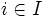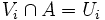# Paracompactness is weakly hereditary

This article gives the statement, and possibly proof, of a topological space property (i.e., paracompact space) satisfying a topological space metaproperty (i.e., weakly hereditary property of topological spaces)
View all topological space metaproperty satisfactions | View all topological space metaproperty dissatisfactions
Get more facts about paracompact space |Get facts that use property satisfaction of paracompact space | Get facts that use property satisfaction of paracompact space|Get more facts about weakly hereditary property of topological spaces

## Statement

### Property-theoretic statement

The property of being a paracompact space is a weakly hereditary property of topological spaces.

### Verbal statement

Any closed subset of a paracompact space is a paracompact space with the subspace topology.

## Proof

Given: A paracompact space$X$, a closed subset$A$ of$X$.

To prove: Consider an open cover of$A$ by open sets$U_i$ with$i \in I$, an indexing set. The$U_i$ have a locally finite open refinement.

Proof:

1. By the definition of subspace topology, we can find open sets$V_i$ of$X$ such that$V_i \cap A = U_i$, thus the union of the$V_i$s contains$A$.
2. Since$A$ is closed, we can throw in the open set$X \setminus A$, and get an open cover of the whole space$X$.
3. Since the whole space is compact, this open cover has a locally finite open refinement. In other words, there is a locally finite open refinement of the$V_i$s, that, possibly along with$X \setminus A$, covers the whole of$X$.
4. By throwing out any member of this new refinement that do not intersect$A$, we get a locally finite open refinement of$V_i$s whose union contains$A$. The intersections of these with$A$ form a locally finite open refinement of the$U_i$s: The main point here is that if an open set in the refinement is contained in$V_i$, its intersection with$A$ is contained in$U_i$.